# Approximation and Interpolation of Functions

The following article is from The Great Soviet Encyclopedia (1979). It might be outdated or ideologically biased.

## Approximation and Interpolation of Functions

the branch of the theory of functions devoted to the study of problems in the approximate representation of functions.

The approximation of a given function f involves the finding of a function g from a certain specified class—for example, from among algebraic polynomials of a stipulated degree—such that g is close to f in some sense, for example, it is an approximate representation of f Many different versions of the problem of the approximation of functions exist, depending on what functions are used for approximation, how the approximating function g is sought, and what is understood by closeness of the functions f and g. A special case of the approximation problem is the interpolation of functions, where it is required that at certain points, or interpolation nodes, the values of the function f coincide with the values of the approximating function g. In the more general case of interpolation, the values of certain derivatives of the two functions are also required to coincide.

In order to estimate the closeness of the original function f to the approximating function g, metrics of various function spaces are used. Usually, these are the metric for the space C of continuous functions, known as the uniform metric, and the metrics for the spaces Lp of functions with integrable pth power, where ρ ≥ 1. In these spaces the distance between f and g is determined (for functions defined on the interval [a, b]) according to the formulas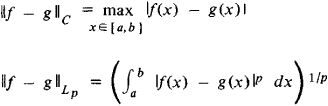The problem most frequently encountered and best studied is that of approximating functions by means of polynomials—that is, by expressions of the form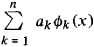where ɸ1, …, ɸn are given functions and a1, …, an are arbitrary numbers. The polynomials are usually algebraic: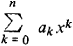or trigonometric: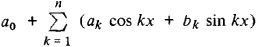Other objects of study include orthogonal polynomials and eigenfunctions of boundary value problems. The rational functions P(x)/Q(x), where algebraic polynomials of a specified degree are taken as P and S, are another classic means of approximation.

Approximation by means of spline functions has been developed in the 1960’s and 1970’s. A characteristic example of splines is the cubic spline function, which is defined as follows. The interval [a, b] is partitioned by the points a = x0 < x1 < … < xn = b. On each interval [xk, xk + 1] the cubic spline function is an algebraic polynomial of degree 3. The polynomials are chosen in such a way that on the interval [a, b] the spline function and its first and second derivatives are continuous. The parameters that remain free can be determined, for example, so that the spline function coincides with the approximated function at the nodes xk. Improvement of the approximation is achieved by increasing the number of nodes xk and correctly arranging them on the interval [a, b]. Spline functions have proven to be convenient in computer mathematics, and they have also been used to solve certain problems in the theory of functions.

The approximation theory of functions, also called the constructive theory of functions, is concerned with approximate representation of functions as well as the study of functions on the basis of their approximate representations. Problems in the approximation of elements in Banach and general metric spaces are usually also included in the approximation theory of functions.

The approximation theory of functions originated with the work of P. L. Chebyshev. He introduced one of the principal concepts of the theory—the concept of the best approximation of a function by polynomials—and he obtained a number of results concerning best approximations. The best approximation of a continuous function f(x) by the polynomials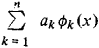relative to the uniform metric is given by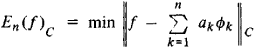where the minimum is taken over all the n-tuples of numbers a1, …, an. The polynomial for which the minimum is attained is called the polynomial of best approximation. The definitions are similar for other metrics. Chebyshev showed that, relative to the uniform metric, the best approximation of the function xn + 1 on the interval [–1, 1] by polynomials of degree n is equal to 1/2n. For the polynomial of best approximation, we have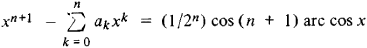The following theorem of Chebyshev indicates a characteristic property of polynomials of best approximation in the space of continuous functions: the algebraic polynomial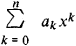is the polynomial of best approximation to the continuous function/on [–1, 1] if and only if there exist n + 2 points, – 1 ≤ x1 < x2 < … < xn + 2 ≤ 1, at which the difference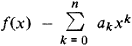takes on the maximum of its absolute value with successively alternating signs. Another early result of approximation theory is the Weierstrass theorem, according to which any continuous function can be uniformly approximated by algebraic polynomials of sufficiently high degree.

Systematic investigation of the behavior, as n → ∞, of the sequence En (f) of best approximation to the function f by algebraic or trigonometric polynomials began early in the present century. The so-called direct theorems of approximation theory express the dependence of the rates at which the values En (f) tend to zero on the properties of the function f The inverse theorems utilize the sequence of best approximations to f to deduce its properties. In a number of important cases, a complete characterization of the properties of functions has been obtained. Two such theorems follow.

In order for the function f to be analytic on an interval—that is, representable at each point of the interval by a power series that converges uniformly to the function in some neighborhood of the point—it is necessary and sufficient that the terms of the sequence of best approximations to the function by algebraic polynomials satisfy the inequalities

En (f)CAqn

where q < ļ and A are certain positive numbers independent of n. This theorem was proved by S. N. Bernshtein.

In order for the function f with the period to have a derivative of order r, r = 0, 1, 2, …, such that

ǀf(r) (x + h) – f(r) (x)ǀ ≤ Mǀhǀα

where 0 < α < 1 and M is some positive number, or such that

ǀf(r) (x + h) – 2f(r) (x) + f(r) (x - h)ǀ ≤ Mǀhǀ

(in this case α = 1), it is necessary and sufficient that the best approximations of f by trigonometric polynomials satisfy the inequalities

En (f)CA/nr+α

where A is some positive number independent of n. The direct theorem here was essentially established by D. Jackson of the USA. The inverse theorem is a result of investigations by Bernshtein and C. J. de La Vallée Poussin and by A. Zygmund of the USA. A characterization of such classes of functions defined on an interval turned out to be impossible in terms of best approximation by algebraic polynomials. It was successfully obtained by resorting to consideration of approximation functions with an improvement of the order of approximation near the ends of the interval.

The possibility of characterizing classes of functions through approximation by polynomials has been made use of in a number of problems in mathematical analysis. In the course of his investigations of best approximation of functions of several variables by polynomials, S. M. Nikol’skii has formulated a theory of imbeddings of some classes of differentiable functions of several variables for classes that are important in analysis. His theory contains not only direct theorems but also inverse theorems that are complete converses of the direct theorems.

A polynomial of best approximation can easily be constructed for approximations relative to the metric L2. For other spaces, the finding of polynomials of best approximation is a difficult problem, and it can be solved only in individual cases. As a result, various algorithms for the approximate determination of polynomials of best approximation have been developed.

One reason for the difficulty of finding polynomials of best approximation is that the operator associating with each function its polynomial of best approximation is not linear: the polynomial of best approximation for the sum f + g is not necessarily equal to the sum of the polynomials of best approximation for the functions f and g. The problem therefore arose of studying linear operators that were as simple as possible and that associated with each function a polynomial yielding a good approximation. For example, for a periodic function f(x) we can take the partial sums of its Fourier series Sn (f, x). According to a theorem of H. Lebesgue, we have the inequality

fSn (f)∥ ≤ (Ln + 1) En (f)C

The Ln are numbers that increase as (4/π2) In n when n → ∞; they have come to be called Lebesgue constants. This bound shows that the polynomials Sn (f) yield an approximation that does not differ greatly from the best approximation. A similar inequality holds for approximations by trigonometric interpolation polynomials with equally spaced interpolation nodes and for approximations by algebraic interpolation polynomials on the interval [–1, 1] with the nodes xk = cos[(2k – 1)/2n]π, k = 1, 2, …, n—in other words, at the zeros of the Chebyshev polynomial cos n arc cos x. For the most important classes of functions encountered in analysis there are linear operators constructed by means of Fourier series or on the basis of interpolation polynomials such that the values of the operators are polynomials that yield for functions in a class the same order of decrease of the approximations when n → ∞ as do the best approximations.

A. N. Kolmogorov began the study of a new problem in the theory of approximations—the problem of the diameter of a class of functions. The problem involves finding for a fixed n a system of n functions ɸ1, …, ɸn such that the best approximations to the functions of a given class by polynomials of the form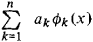would be smallest. It was subsequently found that for a number of important classes of periodic functions the best systems in the sense indicated consist of trigonometric polynomials.

The approximation theory of functions is one of the most active research areas of the theory of functions. The ideas and methods of approximation theory are the starting points for investigations of a number of problems of computer mathematics. Since 1968, a specialized periodical, Journal of Approximation Theory, has been published in the USA.

### REFERENCES

Monographs
Akhiezer, N. I. Lektsii po teorii approksimatsii, 2nd ed. Moscow, 1965.
Goncharov, V. L. Teoriia interpolirovaniia i priblizheniia funktsii, 2nd ed. Moscow, 1954.
Natanson, I. P. Konstruktivnaia teoriia funktsii. Moscow-Leningrad, 1949.
Nikol’skii, S. M. Priblizhenie funktsii mnogikh peremennykh i teoremy vlozheniia. Moscow, 1969.
Timan, A. F. Teoriia priblizheniia funktsii deistvitel’nogo peremennogo. Moscow, 1960.
Surveys
Matematika v SSSR za tridtsat’ let, 1917–1947. Moscow-Leningrad, 1948. Pages 288–318.
Matematika v SSSR za sorok let, 1917-1957, vol. 1. Moscow, 1959. Pages 295-379.
Istoriia otechestvennoi matematiki, vol. 3. Kiev, 1968. Pages 568-88.

S. A. TELIAKOVSKII